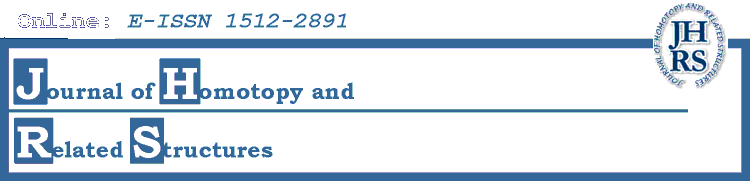# Editors' Interests

• Michael Barr
Category theory, acyclic models and its applications in homological algebra.
• Ronald Brown
Category theory, higher dimensional algebra, holonomy, groupoids and crossed objects in algebraic topology.
• Aurelio Carboni
Categorical methods in algebra, logic and geometry.
• Antonio Cegarra
Category theory, homological and homotopical algebra.
• Claude Cibils
Cohomological multiplicative structures, Representations of quantum groups.
• Frederick Cohen
Algebraic topology, group theory, surface theory, cohomology of groups, applications to physics.
• Guillermo Cortinas
K-theory, cyclic homology, noncommutative geometry.
Operator algebras, K-theory, noncommutative topology.
• Daniel Davis
Stable homotopy theory, spectra with continuous actions by profinite groups, Morava E-theory.
• Peter Eccles
Homotopy theory, cobordism theory and relationship between them.
• Graham Ellis
Homological and homotopical algebra.
Stable homotopy theory, algebraic geometry of formal groups, cohomology of profinite groups.
• Marino Gran
Categorical algebra, Galois theory, universal algebra.
• John Greenlees
Equivariant topology, commutative algebra in topology, representation theory in topology.
• Daniel Guin
Noncommutative geometry.
• Lars Hesselholt
Algebraic K-theory, p-adic arithmetic algebraic geometry.
• Johannes Huebschmann
Homological algebra, algebraic topology, topological methods in physics.
• Hvedri Inassaridze
K-theory, homological and homotopical algebra, noncommutative geometry.
• Nick Inassaridze
Homological and homotopical algebra, K-theory, cyclic homology, algebraic topology.
• Stefan Jackowski
Homotopy theory, classifying spaces, group actions, homological algebra, relationships and applications across various fields of algebra and topology.
• George Janelidze
Category theory, homological algebra, Galois theory.
Homotopy algebra, homotopical physics.
• Pascal Lambrechts
Rational homotopy theory and applications to geometry.
• Ralf Meyer
K-theory and bivariant K-theory, non-commutative geometry, cyclic homology, homological algebra.
• Brian Munson
Algebraic topology, manifolds, Embeddings, immersions, links, calculus of functors, Lie groups.
• Krzysztof Pawałowski
Transformation groups, more specifically, group actions on manifolds.
• Erik K. Pedersen
Algebraic and geometric topology.
• Tim Porter
Algebraic homotopy, homotopy coherence, strong shape theory and proper homotopy theory, global actions, groupoid atlases, abstract homotopy theory.
• Stewart Priddy
Stable homotopy theory.
• Martin Raussen
Applications of homotopy theory in computer science.
• Ulf Rehmann
Linear algebraic groups and related structures.
• Justin Roberts
Topological quantum field theory, low-dimensional topology, quantization, symplectic geometry, homological methods in geometry.
• Jonathan Rosenberg
Topology and geometry of manifolds, index theory, noncommutative geometry.
• Jiri Rosicky
Category theory, homotopy theories, homotopy categories.
• Thomas Schick
Geometric topology, K-theory in particular of operator algebras.
• Ross Staffeldt
Algebraic topology, algebraic K-theory.
• James Stasheff
Higher homotopy algebra, operads, cohomological physics, homotopical physics.
• Ross Street
Enriched category theory, higher-dimensional category theory.
• Guoping Tang
Classical groups, algebraic K-theory, group rings.
• Walter Tholen
Category theory and its applications to algebra, topology and computer science.